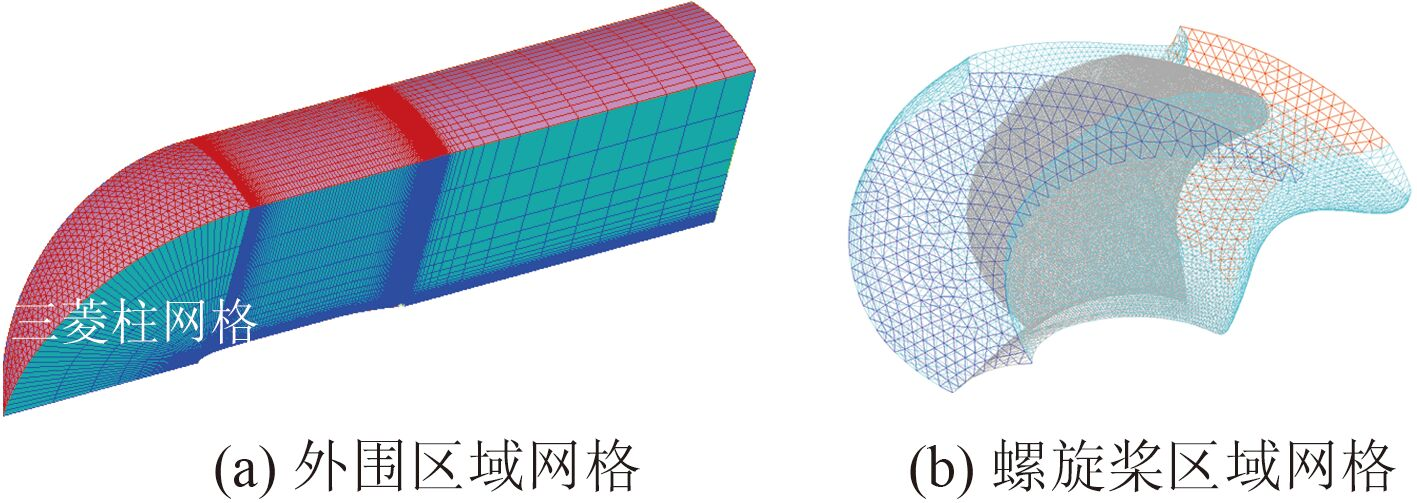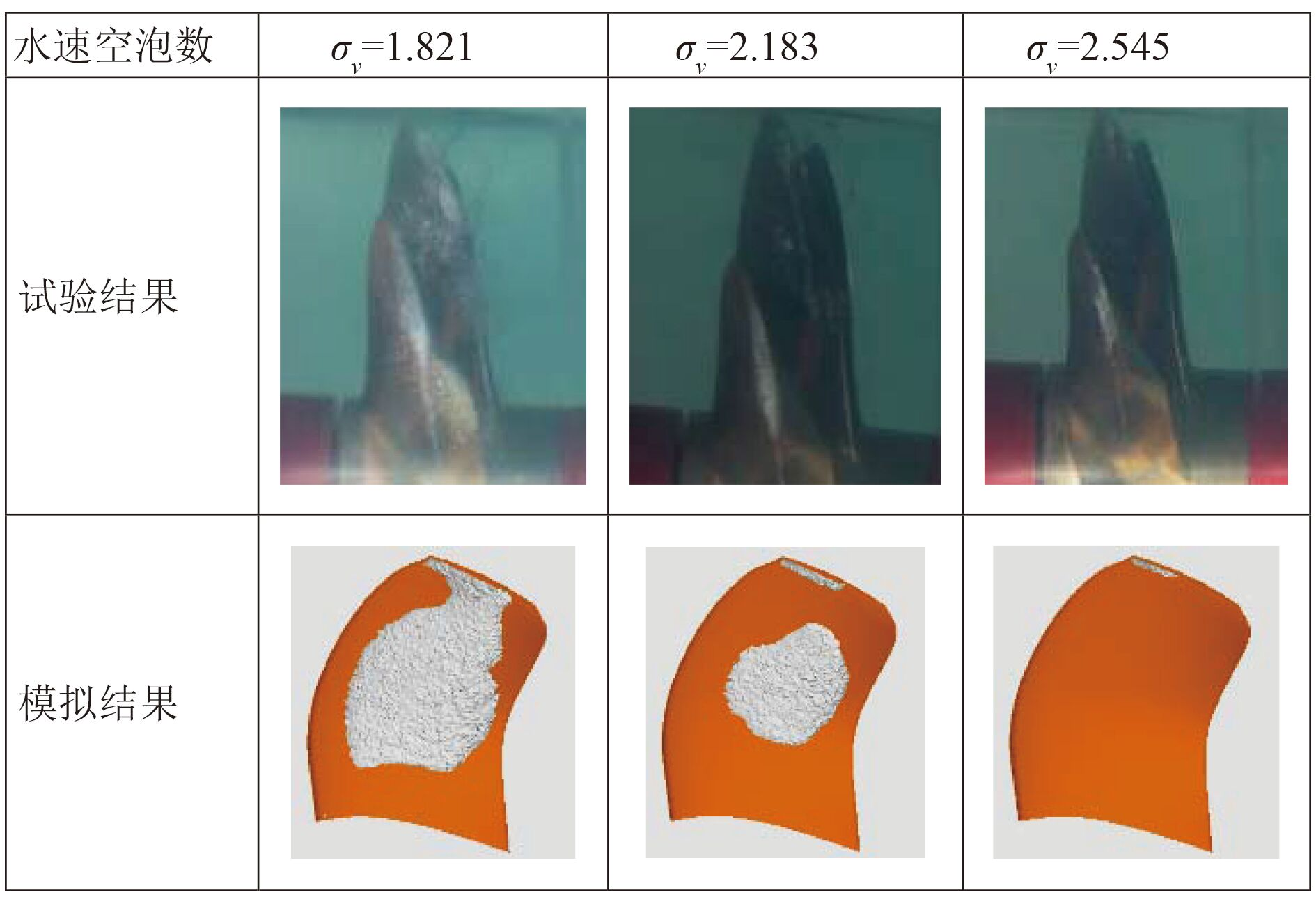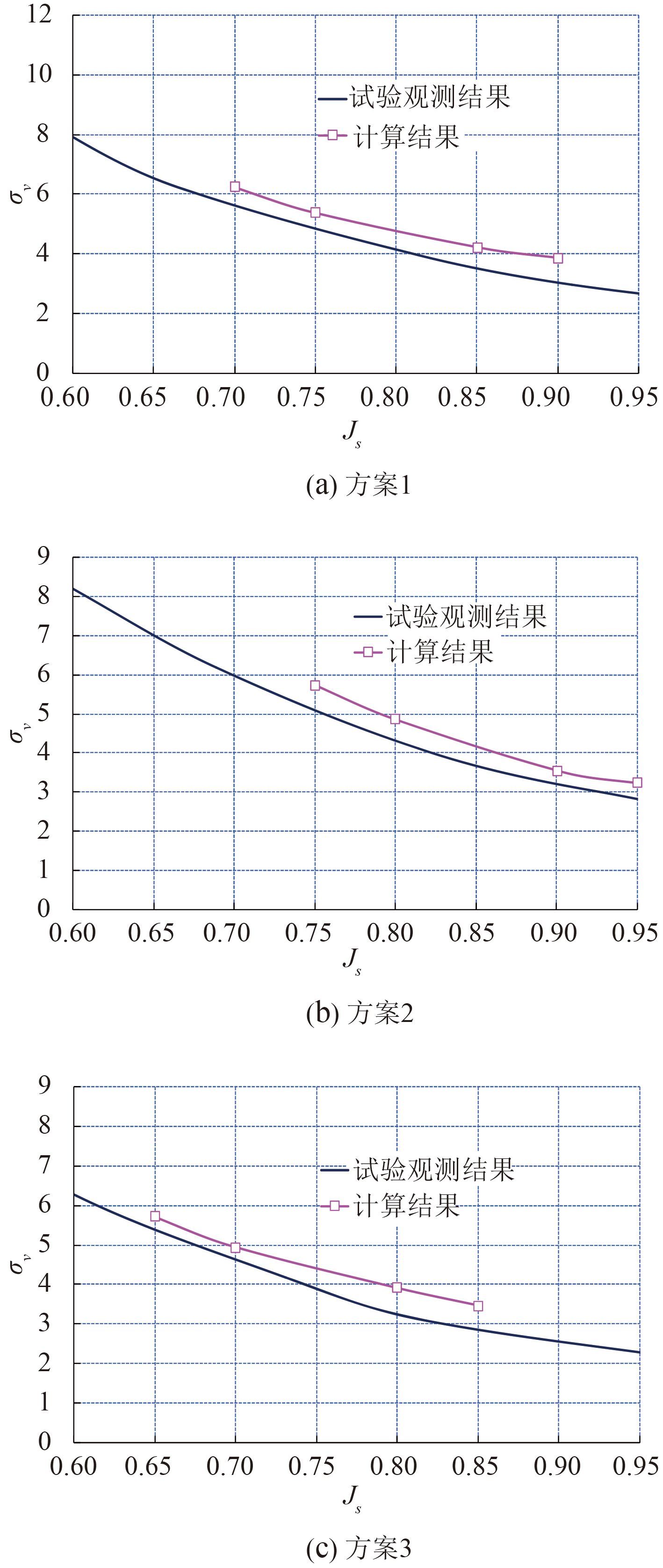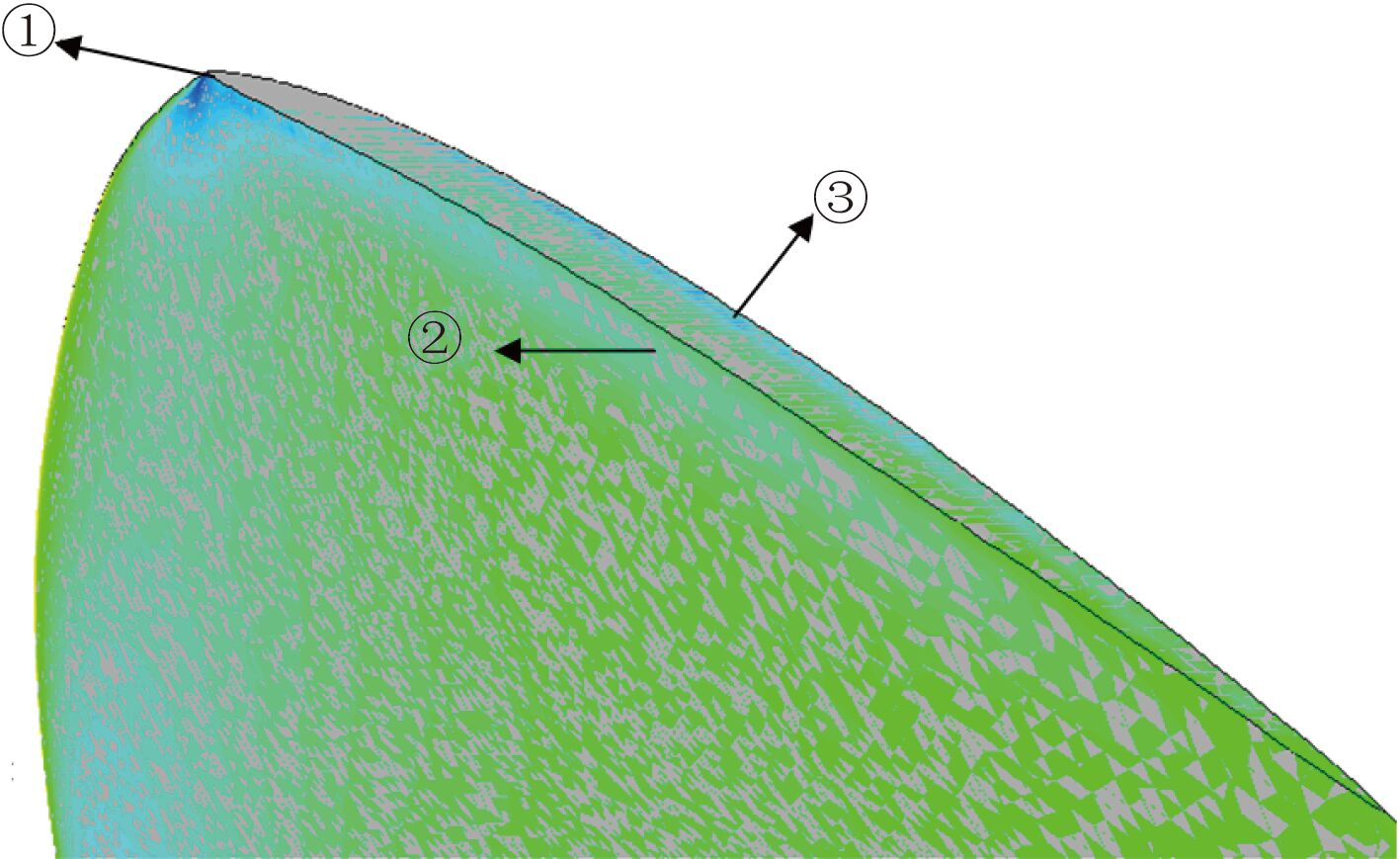﻿ 带前置定子的导管桨片空泡数值预报及梢涡空泡起始判别研究
 舰船科学技术2016, Vol. 38Issue (5): 26-29PDF

1. 海军991办公室, 北京 100841 ;
2. 海军装备研究院, 北京 100161

Research on sheet cavitation numerical predicted and estimation of tip vortex cavitation inception of ducted propeller with pre-swirl stator
FANG Guo-qiang1, QIAN Zheng-fang2, JIANG Jing-wei2
1. 991 Office of Naval, Beijing 100841, China ;
2. Naval Academy of Armament, Beijing 100161, China
Abstract: In the paper, the research on sheet cavitation numerical predicted and estimation of tip vortex cavitation inception of ducted propeller with pre-swirl stator behind underwater vehicle is carried out. Firstly, the sheet cavitation shape of ducted propeller with pre-swirl stator was numerically simulated using a hybrid mesh based on RANS solver. A Singhal cavitation model based on transport equation was coupled in the RANS solver. The predicted sheet cavitation shapes were in good agreement with experimental observation. The overall results suggest that the present approach on predicting sheet cavitation shape of propeller behind underwater vehicle is practicable. Secondly, the wetted flow of ducted propeller with pre-swirl stator behind underwater vehicle is simulated, and the tip vortex cavitation inception is estimation based on the minimum pressure value on the propeller tip section, the results is also in good agreement with experimental results, it show that the approach on estimation of tip vortex cavitation inception is feasible.
Key words: ducted propeller with pre-swirl stator     sheet cavitation     tip vortex cavitation inception     numerical predicted
0 引言

1 数值计算方法 1.1 控制方程

 $\frac{\partial \left( {{\rho }_{m}}{{u}_{i}} \right)}{\partial {{x}_{i}}}=0\text{,}$ (1)
 $\frac{\partial \left( {{\rho }_{m}}{{u}_{i}}{{u}_{j}} \right)}{\partial {{x}_{j}}}=-\frac{\partial p}{\partial {{x}_{i}}}+\frac{\partial }{\partial {{x}_{j}}}\left[ \left( \mu +{{\mu }_{t}} \right)\left( \frac{\partial {{u}_{i}}}{\partial {{x}_{j}}}+\frac{\partial {{u}_{j}}}{\partial {{x}_{i}}} \right) \right]。$ (2)

1.2 空泡模型

 $\frac{1}{{{\rho }_{m}}}=\frac{f}{{{\rho }_{v}}}+\frac{1-f}{{{\rho }_{l}}},$ (3)

 $\frac{\partial \left( {{\rho }_{m}}f \right)}{\partial t}+\nabla \left( {{\rho }_{m}}\overset{\rightharpoonup }{\mathop{V}}\,f \right)=\nabla \left( \Gamma f \right)+{{R}_{e}}-{{R}_{c}}。$ $(4) 式中：源项${R_e}$和${R_c}$分别为汽体生成率和冷凝率，它们均是流场参量（压力、速度）和流体特性（密度、饱和蒸汽压及表面张力）的函数。Singhal.A.K给出源项${R_e}$和${R_c}$的表达式如下： ${{R}_{e}}=-{{C}_{e}}\frac{\sqrt{k}}{S}{{\rho }_{l}}{{\rho }_{v}}{{\left( \frac{2}{3}\frac{{{p}_{v}}-p}{{{\rho }_{l}}} \right)}^{1/2}}(1-{{f}_{v}}-{{f}_{g}})\text{,if}p<{{p}_{v}}\text{;}$(5) ${{R}_{c}}={{C}_{c}}\frac{\sqrt{k}}{S}{{\rho }_{l}}{{\rho }_{l}}{{\left( \frac{2}{3}\frac{p-{{p}_{v}}}{{{\rho }_{l}}} \right)}^{1/2}}{{f}_{v}}\text{,if}p>{{p}_{v}}\text{。}$(6) 式中：${C_e}$和${C_c}$为经验常数，默认值分别为 0.02 和 0.01；k 为湍动能；S 为表面张力；${f_g}$为不可冷凝气体质量分数；${f_v}$为汽体质量分数。计入湍流脉动影响后的临界饱和蒸汽压为： ${{p}_{v}}={{p}_{sat}}+\frac{1}{2}{{p}_{turd}}\text{。}$(7) 式中：${p_{turb}} = 0.39\rho k$；${p_{sat}}\$ 为饱和蒸汽压。

1.3 网格生成图 1 计算网格示意图 Fig. 1 Sketch map of computational mesh
2 片空泡预报结果及试验对比图 2 螺旋桨片空泡模拟结果与模型试验结果对比 Fig. 2 The comparison of propeller sheet cavitation between predicted results and model test results

3 梢涡空泡起始判别图 3 螺旋桨梢涡空泡起始预报结果与模型试验结果对比 Fig. 3 The comparison of propeller tip vortex cavitation inception between predicted results and model test results表 1 三个方案起始空泡数预报结果与试验结果的比较 Tab.1 The comparison of cavitation inception between predicted and model test results图 4 螺旋桨叶梢最小负压出现位置
4 结语

  MCCORMICK B W. On cavitation produced by a vortex trailing from a lifting surface[J]. Journal of Basic Engineering , 1962, 84 (3) :369–378. DOI:10.1115/1.3657328  BILLET M L, HOLL J W. Scale effects on various types of limited cavitation[C]//Proceedings of the International Symposium on Cavitation Inception, the ASME Winter Annual Meeting. New York, NY, USA:ASME, 1979.  CHOW J, ZILLIAC G, BRADSHAW P. Turbulence measurements in the near field of a wingtip vortex[C]//Proceedings of the ASME forum on turbulence in complex flows, Chicago, Illinois, USA. Washington, DC:National Aeronautics and Space Administration, 1997.  FRUMAN D H, CERRUTTI P, PICHON T, et al. Effect of hydrofoil planform on tip vortex roll-up and cavitation[J]. Journal of Fluids Engineering , 1995, 117 (1) :162–169. DOI:10.1115/1.2816806  SENOCAK I, SHYY W. Numerical simulation of turbulent flows with sheet cavitation[C]//Proceedings of the 4th international symposium on cavitation. Pasadena, CA, USA:California Institute of Technology, 2001.  SENOCAK I, WEI SHYY W. A pressure-based method for turbulent cavitating flow computations[J]. Journal of Computational Physics , 2002, 176 (2) :363–383. DOI:10.1006/jcph.2002.6992  RHEE S H, KAWAMURA T, LI H Y. A study of propeller cavitation using a RANS CFD method[C]//Proceedings of the 8th International Conference on Numerical ship Hydrodynamics. Busan, Korea:NSH, 2003.  LIU D C, HONG F W, ZHAO F, et al. The CFD analysis of pro-peller sheet cavitation[C]//Proceedings of the 8th international conference on hydrodynamics, ICHD-2008. Nantes, France:ICHD, 2008.  刘登成, 洪方文, 张志荣, 等. 螺旋桨片状空泡的CFD分析[J]. 舰船科学技术 , 2009, 31 (1) :43–46. LIU Deng-cheng, HONG Fang-wen, ZHANG Zhi-rong, et al. The CFD analysis of propeller sheet cavitation[J]. Ship Science and Technology , 2009, 31 (1) :43–46.